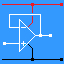RANDOM PAGE

SITE SEARCH

LOG
IN

HELP

# AS ELEC1 / ELEC2

This is the AQA version closing after June 2019. Visit the the version for Eduqas instead.

# AQA A Level

## ELEC1

### Introductory Electronics

#### System Synthesis

• Systems and Subsystems
input
input transducers
process
process subsystems
output
output transducers
feedback ( sometimes )
• System Diagrams
process boxes
lines represent information flow
analysis of whole systems into major subsystems
analysis of subsystems into simpler subsystems

#### Signals

• Signals
analogue
digital

#### Resistive Transducers

• Switch        ON: R = 0        OFF: R = ∞
Digital output
COM = Common
NO = Normally open
NC = Normally closed
• LDR
Log graph scales
Current limiting resistor
Voltage divider
Analogue Output
• Thermistors
Log graph scales
Current limiting resistor
Voltage divider
Analogue Output
Negative temperature coefficient
• Potentiometer
• Voltage divider
Analogue Output
output is proportional to angle or position

#### Operational amplifiers ( op amps )

• Summary
• Ideal Op Amp
Inverting
Non-inverting input
Power supply requirements
Output voltage swing limitations ( ideal and real )
Saturation
• Comparator
Analogue Input
One Bit Digital Output
Analogue to Digital Converter

## ELEC2

### Further Electronics

#### Capacitors

• Capacitors
store energy in the form of charge
block a direct current
allow the passage of an alternating current
unit of capacitance is the Farad
usually measured in pF, nF and µF
working voltage
polarisation ( electrolytic and tantalum capacitors )
leakage current
• In Series       CT = 1 / ( 1 / C1 + 1 / C2 + 1 / C3 + ... )
• In Parallel    CT = C1 + C2 + C3 ...

#### Resistor Capacitor Timing Circuits

• The Time Constant and RC Timing Circuits
T = R C
V = 0.63Vs ( charging )    Vs is the supply voltage
V = 0.37Vs ( discharging )    Vs is the supply voltage
50% Charge or Discharge
T = 0.69 R C
100% Charge or Discharge
Tt = 5 R C
sketch voltage / time graphs for charging and discharging

#### 555 Timer

• Inside the 555
• Monostable
circuit diagram
One stable state
T = 1.1 R C
How it works
• Astable
circuit diagram
No stable states
How it works
Low Time:     tL = 0.7 RB C

High Time:     tH = 0.7 (RA + RB) C

Frequency:     f = 1.44 / (RA + 2 RB ) C

#### Sequential Logic

• bistable latch based on NAND gates
operation
function
• D-type flip-flop
symbol
operation
function
• Shift Register
circuit diagram
operation
applications
• Counters - Up to 4 bits
Feedback to make a D-type flip-flop divide by 2
Up counter
Down counter
Modulo-N counters
Timing diagrams

#### Number Systems

convert a 4-bit binary number to decimal
convert a 4-bit binary number to hexadecimal
Binary Coded Decimal ( BCD )
BCD decoder for a seven segment display

#### Operational Amplifiers

• ideal op-amp properties
Input resistance = ∞
Output resistance = zero
Gain Bandwidth Product
The product of voltage gain and bandwidth is a constant
• Gain = Vout / Vin
• Voltage bandwidth is the frequency range where Vout is at least 70% of the maximum
• Power bandwidth is the frequency range where Vout is at least 50% of the maximum
• inverting amplifier
circuit
Vout = - Vin Rf / Rin
Gain = - Rf / Rin
input resistance = Rin ( usually a low value )
the inverting input is a Virtual Earth
• summing amplifier
circuit
Vout = - Rf ( V1 / R1 + V2 / R2 + V3 / R3 )
input resistance = Rin ( for each input )
the inverting input is a Virtual Earth
• difference amplifier
circuit
Vout = ( V+ - V- ) Rf / R1
input resistance = 2 R1 ( usually a low value )
• non-inverting op-amp amplifier
circuit
Vout / Vin = 1 + Rf / Rin
input resistance is equal to that of the op-amp
• voltage follower
circuit
applications
voltage gain = 1
current and power gain can be very large
input resistance is equal to the resistance of the op-amp
• SUMMARY / Key Facts

#### Power Amplifiers

• Power bandwidth the frequency range where Powerout is at least 50% of the maximum
• Power gain = Pout / Pin
• MOSFET source followers ( N and P Channel )
estimate the power dissipated in a source follower
methods for removing the excess heat
• Heat Sinks
Conduction - use a metal like aluminium
Convection - have a large surface area to let the heated air rise
• Push Pull Amplifier - MOSFET - BJT
Estimate the maximum power output
Compare with a Class A single ended circuit
Cross over distortion is reduced by
biasing the MOSFETs with diodes and resistors
including the MOSFETS in the negative feedback path
Clipping, Limiting, Saturation
The output is limited by the power supply voltage
Increase the voltage but don't exceed the MOSFET ratings.

reviseOmatic V3     Contacts, ©, Cookies, Data Protection and Disclaimers Hosted at linode.com, London